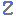Visit Zacobria Webshop

Speedl and pose_trans example:

Application Description:
The speedl command moves the robot in cartesian coordinate system, either moving individual axis or by moving multiple axis at the same time with the option of setting the acceleration and time of the move.

speedl is using the format shown below with each 6 axis as an element in a list where the value is the tool speed. And then an option for the acceleration of the move and the time of the move.

speedl([X, Y, Z Rx, Ry, Rz], accl, time)

pose_trans is using the format shown below where variable “var_1” is transformed by adding “var_2” in tool space.

pose_trans(var_1,var_2)

I/O table Inputs:
N/A

Variable Table:
var_1 = Get the actual pose position.
var_2 = List variable with the value to turn the Rz axis with the tool speed of -0.5
var_3 = The result of transforming var_1 with var_2 in tool space.

Electrical diagrams.
N/A

Function description:

In this example the robot is put in the position as shown below.

Program description:

The first waypoint is the starting of position of the robot in this example to return to after each program run.

The second line moves the tool head in X direction with a tool speed of 0.1 with acceleration 1.0 for 0.5 second.

Then the robot stops and wait for 1 second.

Then the robot move the tool head is all 6 axis with a tool speed of 0.1 with acceleration 1.0 for 0.5 second.

Then the robot stops and wait for 1 second.

Then the robot move the tool head in Rz direction with a tool speed of 0.1 with acceleration 1.0 for 0.5 second.

Thereafter the current robot pose is inserted into var_1.

var_2 is assigned the list value where all elements a 0 except Rz which is set to -0.5 in order to add this value to the current pose in the next line.

var_3 is assigned the value of var_1 transformed (added) with the value of var_2 in tool space.

Finally the robot moves to the transformed position which is a Rz turn at the position where the robot is currently at.

Program code:

Program run:
The robot moves through the 3 positions assigned by using speedl and trans_pose functions and return to start position at each new run.

Notes:
Notice there is a difference in the orientation of the Z axis – whether it is seen with the robot Base as reference – or if it is seen with the robot Tool head as reference. And the actual robot position (current robot pose) also has an influence on how many joints are turning in order to make the move around the Z axis using the Rz parameter.
If the tool head is pointing straight down:
Then if the robot is turning around the Z axis using the Rz parameter with the Base as reference – then only the last joint is turning because the Toolhead is in line with the Robot base Z direction which is straight (vertically) up and down.
But if the tool head is in an angle:
Then if the robot is turning around the Z axis using the Rz parameter with the Base as reference – then several joints might turn – because the TCP point is turning around the Z axis – which might be a big circle in order to follow around the vertical Z axis which is still straight up/down. It is the TCP point that is following the Z axis when using the “Speedl” command.

Instead if the robot is to turn around the Tool heads Z axis then the command “pose_trans” is used.

Disclaimer: While the Zacobria Pte. Ltd. believes that information and guidance provided is correct, parties must rely upon their skill and judgement when making use of them. Zacobria Pte. Ltd. assumes no liability for loss or damage caused by error or omission, whether such an error or omission is the result of negligence or any other cause. Where reference is made to legislation it is not to be considered as legal advice. Any and all such liability is disclaimed.

If you need specific advice (for example, medical, legal, financial or risk management), please seek a professional who is licensed or knowledgeable in that area.

Author:
By Zacobria Lars Skovsgaard
Accredited 2015-2018 Universal Robots support Centre and Forum.

Tweet